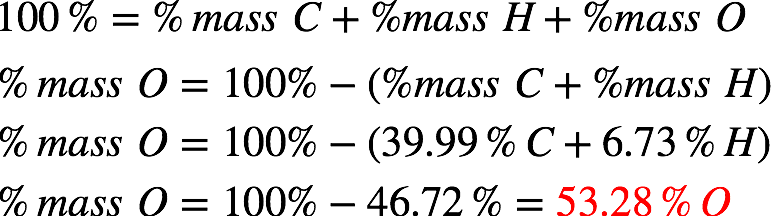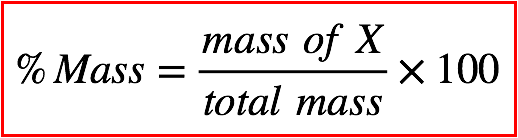# Problem: An organic compound contains C, H, and O. The percentages of H and C are 6.73% H, and 39.99% C by mass. The molar mass is 60.06 amu. What is the molecular formula of the compound?A) CH2OB) C4H8O4C) C2H3O2D) C2H4O2E) C3H6O3

###### FREE Expert Solution
91% (253 ratings)
###### FREE Expert Solution

We’re being asked to determine the molecular formula of a compound composed of C, H, and O given the mass percent of C and H. This means we need to do the following steps:

Step 1: Calculate the mass and moles of C, H, and O in the compound.

Step 2: Determine the lowest whole number ratio of C, H, and O to get the empirical formula.

Step 3: Get the ratio of the molar mass and empirical mass to determine the molecular formula.

Step 1: The compound is composed of C, H, O and we’re only given the mass percent of C (39.99% C) and H (6.73% H). Recall that the mass percentages of a compound must add up to 100%. This means:Recall that mass percent is given by:Assuming we have 100 g of the compound, this means we have 39.99 g C, 6.73 g H, and 53.28 g O. Now, we need to get the moles of each element in the compound. The atomic masses are 12 g/mol C, 1 g/mol H, 16 g/mol O.

91% (253 ratings)###### Problem Details

An organic compound contains C, H, and O. The percentages of H and C are 6.73% H, and 39.99% C by mass. The molar mass is 60.06 amu. What is the molecular formula of the compound?

A) CH2O

B) C4H8O4

C) C2H3O2

D) C2H4O2

E) C3H6O3

What scientific concept do you need to know in order to solve this problem?

Our tutors have indicated that to solve this problem you will need to apply the Molecular Formula concept. You can view video lessons to learn Molecular Formula. Or if you need more Molecular Formula practice, you can also practice Molecular Formula practice problems.

What is the difficulty of this problem?

Our tutors rated the difficulty ofAn organic compound contains C, H, and O. The percentages of...as medium difficulty.

How long does this problem take to solve?

Our expert Chemistry tutor, Dasha took 9 minutes and 32 seconds to solve this problem. You can follow their steps in the video explanation above.

What professor is this problem relevant for?

Based on our data, we think this problem is relevant for Professor N/A's class at Ryerson University.